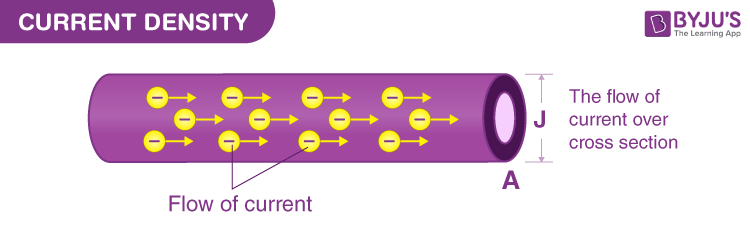# Current Density

We know that the invention of electric current revolutionised living and lifestyle. It is the fundamental source to power many devices like fans to complex devices like boilers and heaters. Current is defined as the flow of electrical charge carriers. In this article, let us learn what is current, types of current, formula, and current density along with solved examples.## Current Definition:

We can define current as the flow of electrically charged particles. The standard symbol of current is capital I. The standard unit of current is ampere and it is denoted by A. Conversely, a current of one ampere is one coulomb of charge (6.24 x 1018 charge carriers) going past a given point per second. According to Physicists, Current is considered to move from relatively positive to negative points, and this is known as conventional current. Electrons are known to be the common negatively-charged carriers and circulate from relatively negative to positive points. In this article, we will learn about the concepts of Current Density in a detailed manner.

## Types of Current

The current can be divided into two types.

### Direct Current:

• Direct current travels in the same direction at all points, although the instantaneous magnitude can differ.
• An example of DC is the current generated by an electrochemical cell.

### Alternating Current:

• The flow of charge carriers is towards the opposite direction periodically in an alternating current.
• The number of AC cycles per second is known as frequency and calculated in Hertz.

## To know more about the current flow in detail, click on the video below## What Is Current Density?

The amount of electric current traveling per unit cross-section area is called as current density and expressed in amperes per square meter. The more the current in a conductor, the higher will be the current density. However, the current density alters in different parts of an electrical conductor and the effect takes place with alternating currents at higher frequencies.

Electric current always creates a magnetic field. Stronger the current, the more intense the magnetic field. Varying AC or DC creates an electromagnetic field and this is the principle based on which signal propagation takes place.

Current density is a vector quantity having both a direction and a scalar magnitude. The electric current flowing through a solid having units of charge per unit time is calculated towards the direction perpendicular to the flow of direction.

It is all about the amount of current flowing across the given region.

## Current Density Formula

The formula for Current Density is given as,

### J = I / A

Where,

I = current flowing through the conductor in Amperes

A = cross-sectional area in m2.

Current density is expressed in A/m2.

## Solved Problem on Current Density

Determine the current density when 40 amperes of current is flowing through the battery in a given area of 10 m2.

Solution:

It is given that,

I = 40 A,

Area = 10 m2

The current density formula is given by,

J = I / A

= 40 / 10

J = 4 A/m2.

## Frequently Asked Questions – FAQs

Q1

### What is current?

We can define current as the flow of electrically charged particles travelling. Electric current is represented as I.
Q2

### What are the types of current?

The following are the types of electric current:

• Direct Current
• Alternating Current
Q3

### What is meant by current density?

The amount of current travelling per unit cross-section area is known as current density and expressed in amperes per square metre.

Q4

### Give the formula for the current density.

The formula for current density is J = I/A.
Q5

### Which type of current is generated by an electrochemical cell?

Direct current is generated by an electrochemical cell.